STL vector练习 转

（一）：vector练习，实现几个排序算法

//================================
// Name : VectorTest.cpp
// Author : hongboChen
// Version :
// Description : 由于上一节学习使用了vector,
// 所以现在练习使用一下vector的使用
// 实现了几个排序的算法
//===============================

#include <iostream>
#include <vector>

using namespace std;

void swap(int &a,int &b);
vector<int>  bubbleSort(vector<int> vec);
vector<int> selectSort(vector<int> vec);
void quickSort(vector<int> &vec,int first_lc,int last_lc);

int main() {

//用于保存vector的大小
int size = 0;

cout << "Please enter the number of the array: ";
cin >> size;

//新建一个vector对象,并设置其大小
vector<int> vec(size);

//获取vector的遍历器
//vector<int>::iterator it = vec.begin();

//获取用户输入的数值,并赋值给vector
cout << "Please enter the value of the array:" <<endl;

for(int i = 0; i < size;i++){
cin >> vec[i];
//或者是
//cin >> vec.at(i);
//或者是
//it += i;
//cin >> *it;
}

//下面就是对数组进行排序
//冒泡排序
//vec = bubbleSort(vec);

//选择排序
//vec = selectSort(vec);

//快速排序
quickSort(vec,0,size-1);

for(int i = 0;i < size;i++){
cout << vec[i] << " ";
}

cout << endl;

return 0;
}

//最简单的就是冒泡排序
/** * 冒泡排序的思想就是 * 每次循环的时候都是找相邻的两个元素 * 如果相邻的两个元素符合顺序要求,就i不必切换位置 * 如果相邻的两个元素不符合顺序要求,就将这两个元素的位置对换 */
vector<int>  bubbleSort(vector<int> vec){
int size = vec.size();

//防止数组越界发生错误
for(int i = 0;i < size-1;i++){
for(int j = i;j < size-1;j++){
if(vec[j] > vec[j+1])
swap(vec[j],vec[j+1]);  //进行交换
}
}

return vec;
}

/** * 下面是选择排序 * 选择排序的算法思想是: * 首先从所有的元素中选出最大的一个元素放在数组最后 * 接着从剩下的元素中再选出最大的一个元素放在后面 * 以此类推,就可以排出顺序 */
vector<int> selectSort(vector<int> vec){

//用于保存选出的元素应该放到哪一个位置当中
int k = vec.size()-1;

for(int i = 0;i < vec.size();i++){
int max_lc = 0;
for(int j = 0;j <= k;j++){
if(vec[j] > vec[max_lc])
max_lc = j;
}

swap(vec[k],vec[max_lc]);
k--;
}

return vec;
}

/** * 快速排序思想:算法复杂度为O(nlog(n)) * * 1:从数组中选出一个元素,通常情况下是选择第一个元素,称为"基准" * 2:将所有的比"基准"小的元素放在基准左边,比"基准大的元素"放在基准右边 * 3:采用递归,排序完成 */
/** * vec : 要排序的数组 * first_lc : 第一个元素的位置 * last_lc : 最后一个元素的位置 * */
void quickSort(vector<int> &vec,int first_lc,int last_lc){

if(first_lc >= last_lc)
return;

int i = first_lc;
int j = last_lc;

int key = vec[first_lc];

while(i < j){

while(i < j && vec[j] > key)
j--;
vec[i] = vec[j];

while(i < j && vec[i] < key)
i++;
vec[j] = vec[i];
}

vec[i] = key;

quickSort(vec,0,i-1);
quickSort(vec,i+1,last_lc);
}

/** * 先设定一个交换函数 */
void swap(int &a,int &b){
int temp = a;
a = b;
b = temp;
}

（二）：快速排序的原理

1:从数组中选出一个元素,通常情况下是选择第一个元素,称为”基准”
2:将所有的比”基准”小的元素放在基准左边,比”基准大的元素”放在基准右边
3:采用递归,排序完成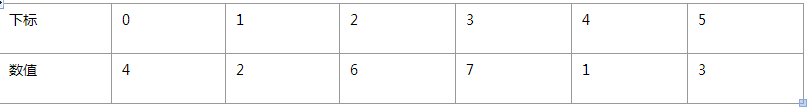1：我们需要定义两个数值：
int i = 0; //第一个元素的位置
int j = 5; //最后一个元素的位置

int key = array[i];

2：由于第一个元素即基准值被key取走了，所以我们需要找一个元素来取代这个位置，

4的元素，在寻找过程中，j不断的在减小。

i = 0;
j = 5;
key = 4;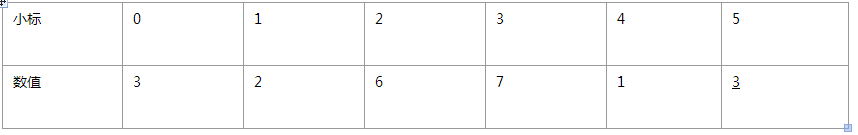3：此时，位置5处的数值3被空出来了，同理，由于需要将大于基准值得元素放在右边，所以我们需要

i = 2;
j = 5;
key = 4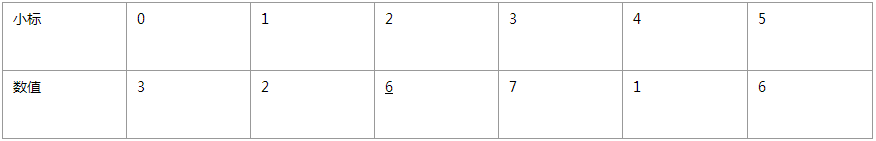4：此时，位置2处的6被空出来了，我们再从j位置开始，从右向左寻找第一个一个小于基准值的元素，

i = 2;
j = 4;
key = 4;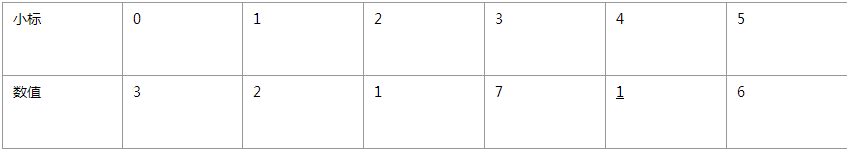5：我们接着再从i位置开始从左向右寻找第一个大于4的元素，就是7，则此时
i = 3;
j = 4;
key = 4;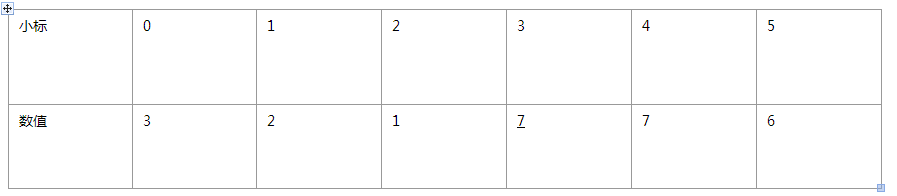array[i] = key;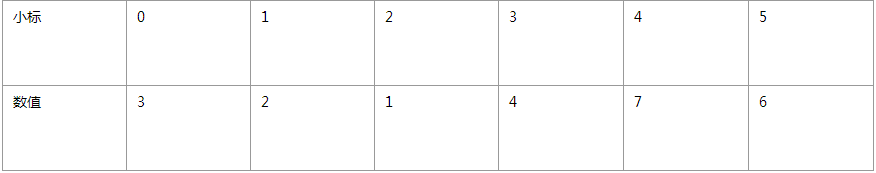C++ STL编程轻松入门 4

2.2.2 第二版：工业时代--组件化大生产 　　我们应该庆幸自己所生活的年代。工业时代，科技的发展所带来的巨大便利已经影响到了我们生活中的每个细节。如果你还在以原始人类的方式生活着，...

2015/11/21
84
0
STL vector 介绍连载1-2-3

STL简介: STL = Standard Template Library，标准模板库，惠普实验室开发的一系列软件的统称。它是由Alexander Stepanov、Meng Lee和David R Musser在惠普实验室工作时所开发出来的。这可能是...

2012/05/20
152
0
C++ STL学习——vector

chenyufeng1991
2016/08/21
0
0
Effective STL - 容器

STL（standard template library）提供了一组表示容器，迭代器，函数对象和算法的模板。容器是一个与数组类似的单元，可以存若干个值。 STL容器是同质的，即存储的值的类型相同；算法是完成特...

2013/10/07
148
0
C++ Primer 学习笔记（第三章：字符串、向量和数组）

C++ Primer 学习笔记（第三章：字符串、向量和数组） [TOC] 3.1 命名空间的声明 声明语句可以一行放多条。 位于头文件的代码，一般来说不应该使用声明。因为其内容会拷贝到每个使用该头文件的...

ShawnLue
2015/08/20
152
0

lianbang_W
37分钟前
3
0
JVM -- Java堆结构及对象分代

Hello，今天记录下 Java虚拟机中的其中一个重点知识 --> Java堆。 一起学习，一起进步。继续沉淀，慢慢强大。希望这文章对您有帮助。若有写的不好的地方，欢迎评论给建议哈！ 初写博客不久，...

43分钟前
4
0
elastic-job的使用

44分钟前
4
0

SKILL语言是Candence提供给用户的一个开发接口，利用其本身提供的接口函数和SKILL语言完成自动化操作的功能。 怎么查看SKILL： 1.可以直接用写字板打开进行编辑或看功能说明。 2.想自己写或改...

demyar
45分钟前
4
0

47分钟前
4
0